In :
import pandas as pd
from __future__ import division
# this enables the 'toggle input' button
from display import extended_styles
from functools import partial
extended_styles(css=True)

Out:
Toggle Input
In :
# read in the data file that has "GOD" and "WORDSUM" variables
# data was gathered from: http://sda.berkeley.edu/sdaweb/analysis/?dataset=gss12
# DATA IS UNWEIGHTED
# na_values defines values of GOD and WORDSUM that are invalid codes
# invalid codes are defined as 0,8,9 for GOD (see GSS codebook)
#    note: I did find some 10's for GOD, and treated them as invalid/missing
# invalid codes are defined as -1,98,99 for WORDSUM

fname = "sub-data-by-year.txt"

# create a data set that has only valid cases
actData = data.dropna()

# make the IQ variable
# (from http://aeolipera.wordpress.com/2013/02/13/conversion-table-from-wordsum-to-iq/)
actData["IQ"] = 15*((actData.WORDSUM - 5.965)/2.148) + 100


#### Data Description

Data source: GSS 1972-2012

Accessed from: http://sda.berkeley.edu/sdaweb/analysis/?dataset=gss12

Variables used: GOD, WORDSUM, YEAR (no weighting)

Invalid codes for each variable:

GOD: [0,8,9,10]

NOTE: The value given for 8 is "DK", which might indicate agnosticism, but that conflicts in some ways with answer 2. However, only 3 values for WORDSUM == 0 are found in the "DK" answers, which won't alter the data much

WORDSUM: [-1,98,99]

#### Histograms of WORDSUM, grouped by GOD

In :
# plot histograms of WORDSUM for each GOD answer
# Histograms for IQ will look the same, since the transformation from WORDSUM to IQ is linear
# Also, the discrete WORDSUM values will result in discrete IQ values, with the same bin counts
# This is over all years in the dataset
#actData.WORDSUM.hist(by=actData.GOD,bins=range(0,11),figsize=(11,8),normed=True,sharey=True);
fig = pylab.figure(figsize=(11,8))
for i in range(0,6):
d = actData.loc[actData.GOD == i+1,"WORDSUM"]
ax.hist(d,bins=range(0,11),normed=True,align="right")
ax.set_ylim(0,.30)
ax.set_xlim(-0.5,10.5)
ax.set_xticks(range(0,11))
ax.set_title("GOD = %d"%(i+1))
ax.text(0,.225,"Number of data points = %d"%len(d))
#ax.set_xlabel("WORDSUM")
if not i in [0,2,4]:
ax.set_yticklabels([])
else:
ax.set_ylabel("Frequency")
if not i in [4,5]:
ax.set_xticklabels([])
else:
ax.set_xlabel("WORDSUM")
fig.tight_layout()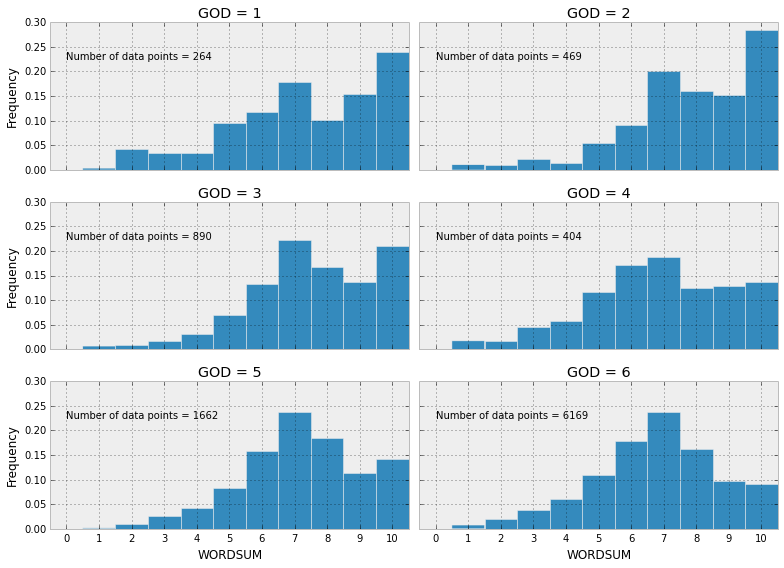#### IQ Transformation

Uses the transformation from Aeoli Pera:

$$IQ = 15 \frac{V - 5.965}{2.148} + 100$$

IQ vs WORDSUM scores are given below, for each answer in GOD:

In :
# get IQ data for plotting
iqVals = *6
print "Total Number of Data Points: %d"%len(actData)
for i in range(1,7):
iqVals[i-1] = actData.loc[actData.GOD == i, "IQ"].value_counts()
print "Number of data points for God = %d : %d"%(i,sum(iqVals[i-1]))
# convert to frequency, then percentage
iqVals[i-1] /= iqVals[i-1].sum()
iqVals[i-1] = iqVals[i-1]*100
# sort for easy plotting
iqVals[i-1] = iqVals[i-1].sort_index()

# plot
fig = pylab.figure(figsize=(10,7))
for i in range(0,6):
ax.plot(iqVals[i].index,iqVals[i],label = "GOD = %d"%(i+1))
ax.set_ylim(0,30)
ax.set_ylabel("Percentage")
ax.set_xlabel("IQ")
ax.legend(loc="best")
pylab.title("Comparison of IQ Values\nGSS 1972-2012");

Total Number of Data Points: 9858
Number of data points for God = 1 : 264
Number of data points for God = 2 : 469
Number of data points for God = 3 : 890
Number of data points for God = 4 : 404
Number of data points for God = 5 : 1662
Number of data points for God = 6 : 6169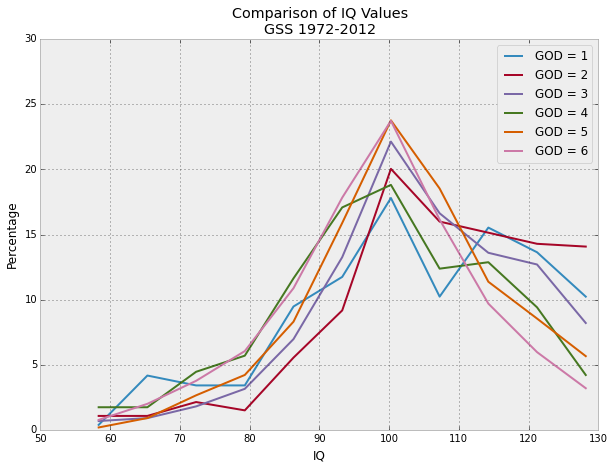Comparing the extremes (1 is atheist, 6 is non-doubting theist):

In :
# plot just two of the values for comparison
fig = pylab.figure(figsize=(10,7))
ax.plot(iqVals.index,iqVals,marker='o',label = "GOD = 1")
ax.plot(iqVals[-1].index,iqVals[-1],marker='o',label = "GOD = 6")
ax.set_ylim(0,30)
ax.set_ylabel("Percentage")
ax.set_xlabel("IQ")
ax.legend(loc="best")
pylab.title("Comparison of IQ Values\nGSS 1972-2012");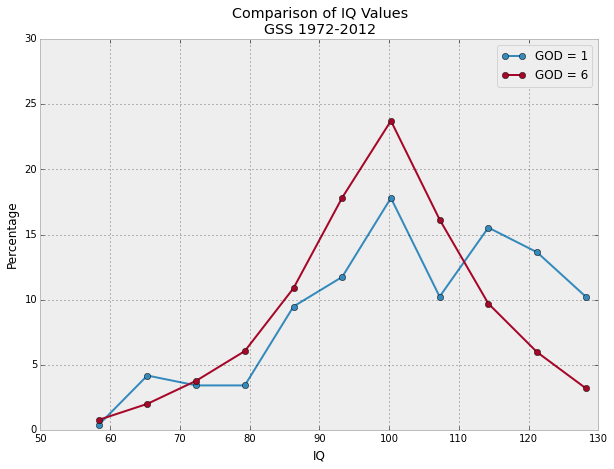Same plots as above, but restricted to only the most recent survey results (2012):

In :
# repeat, but restrict to 2012
# This data will have very few data points, so it's not very reliable
print "Total Number of Data Points: %d"%sum(actData.loc[(actData.YEAR == 2012), "IQ"].value_counts())
# get IQ data for plotting
iqValsByYear = *6
for i in range(1,7):
iqValsByYear[i-1] = actData.loc[(actData.GOD == i) & (actData.YEAR == 2012), "IQ"].value_counts()
print "Number of data points for God = %d : %d"%(i,sum(iqValsByYear[i-1]))
# convert to frequency, then percentage
iqValsByYear[i-1] /= iqVals[i-1].sum()
iqValsByYear[i-1] = iqVals[i-1]*100
# sort for easy plotting
iqValsByYear[i-1] = iqVals[i-1].sort_index()

# plot
fig = pylab.figure(figsize=(10,7))
for i in range(0,6):
ax.plot(iqValsByYear[i].index,iqValsByYear[i],label = "GOD = %d"%(i+1))
ax.set_ylim(0,30)
ax.set_ylabel("Percentage")
ax.set_xlabel("IQ")
ax.legend(loc="best")
pylab.title("Comparison of IQ Values\nGSS 2012");

Total Number of Data Points: 1270
Number of data points for God = 1 : 46
Number of data points for God = 2 : 75
Number of data points for God = 3 : 142
Number of data points for God = 4 : 52
Number of data points for God = 5 : 209
Number of data points for God = 6 : 746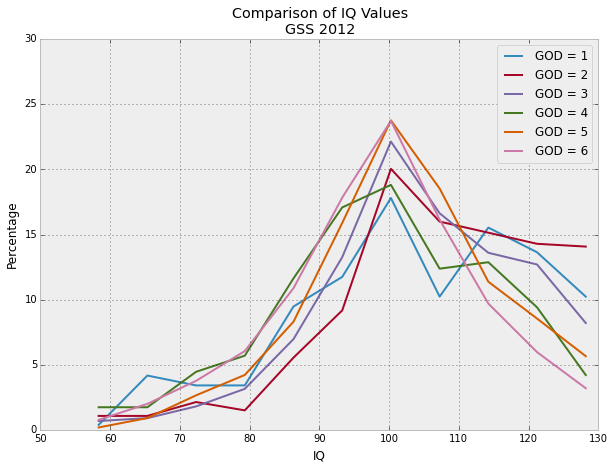In :
# plot just two of the values for comparison
fig = pylab.figure(figsize=(10,7))
ax.plot(iqValsByYear.index,iqValsByYear,marker='o',label = "GOD = 1")
ax.plot(iqValsByYear[-1].index,iqValsByYear[-1],marker='o',label = "GOD = 6")
ax.set_ylim(0,30)
ax.set_ylabel("Percentage")
ax.set_xlabel("IQ")
ax.legend(loc="best")
pylab.title("Comparison of IQ Values\nGSS 2012");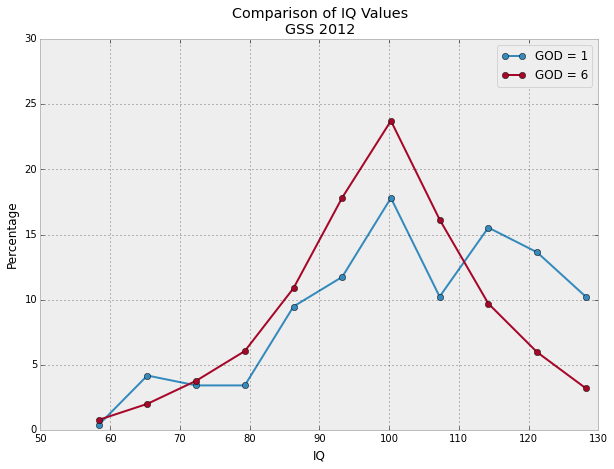In :
# this enables the nice CSS styling
from IPython.core.display import HTML
def css_styling():